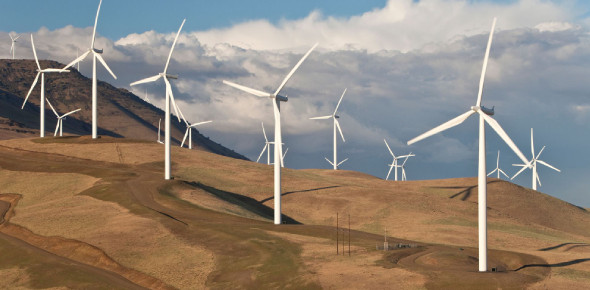# Quiz: How Well You Know About Wind Energy? Trivia

12 Questions | Attempts: 517Settings.

• 1.
How long does it take for a turbine to pay back the electricity used to manufacture it?
• A.

6 months

• B.

8 months

• C.

5 months

• D.

7 months

• 2.
How does a wind turbine make electricity?
• A.

The wind turns a generator inside

• B.

Magic

• C.

Waves turn the generator

• D.

Hamster turn the generator

• 3.
How long do wind turbines last ?
• A.

10-15 years

• B.

35-40 years

• C.

20-25 years

• 4.
How much electricity does one wind turbine produce?
• A.

5,7 million units of electricity

• B.

4,7 million units of electricity

• C.

3,7 million units of electricity

• 5.
Wind Turbines cause high-frequency noise.
• A.

True

• B.

False

• 6.
How much of the time do wind turbines produce electricity?
• A.

75%-80%

• B.

50%-60%

• C.

90%-95%

• 7.
Where is the biggest wind farm in the world?
• A.

Berlin

• B.

• C.

Kent

• 8.
How many wind turbines are there in the UK?
• A.

3730 3730 v3730 3730 3730 3730

• B.

4730

• C.

5730

• 9.
What creates wind?
• A.

Magic

• B.

Sun

• C.

Moon

• 10.
What percentage of people like wind turbines?
• A.

5%

• B.

10%

• C.

15%

• 11.
What percentage of people like wind turbines?
• A.

5%

• B.

10%

• C.

15%

• 12.
How much electricity does one wind turbine produce?
• A.

5,7 million units of electricity

• B.

4,7 million units of electricity

• C.

3,7 million units of electricity

## Related TopicsBack to top
×

Wait!
Here's an interesting quiz for you.